## Posts

Showing posts from July, 2010

### Roots of a Quadratic Equation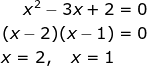What do we mean by the roots of a quadratic equation?

Example 1

We say that 2 and 1 are roots of the equation. The equation has 2 real and distinct roots

In graphical form, the roots of the equation are represented as the intersection of the curve with the x axis.

Example 2

In this case there is only 1 solution. We say that the equation has only 2 real and equal roots
Graphically it can be represented as

Notice that there is only 1 intersection point between the curve and the x axis

Example 3
Lastly we are going to look at an example where the curve does not intersect the x axis
Consider the equation:

The equation cannot be factorized. It cannot be solved using the quadratic formula.
Graphically it is represented as

It turns out that we can predict the number of roots that an equation has by looking at the discriminant

### Remainder theorem II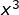Question 1
The coefficient of the term in a cubic polynomial f(x) is -1. The roots of f(x) are 1, 2 and k. f(x) when divided by x - 3 gives a remainder of 8.
Find the value of k

First we need to understand what is meant by roots of an equation

### Remainder theorem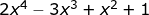Introduction

How do we find the remainder when we divide by ?

One way is to use long division

From the long division, we get a remainder of 13

Note that:
is known as the divisor
is known as the quotient
is known as the dividend

In most cases we are only interested in the remainder, there is an easier way of obtaining the remainder without using long division

The easier way is to use the Remainder Theorem

Once again we want to find the remainder when is divided by

How do we know what value to sub in ?
If we are dividing by x - 2, we let x - 2 =0 and get x = 2. So we sub in 2
If we are dividing by x + 2, we let x + 2 =0 and get x = -2. So we sub in -2
If we are dividing by x - a, we let x - a =0 and get x = a. So we sub in a

Question 1
Given that leaves a remainder of 6 when divided by and has a factor of , find the value of a and b.

Next the question says that x + 2 is a factor
Factor means that the remainder is zero
Hence we can apply the remainder theorem

By solving the 2 simultaneous equat…

### Binomial Theorem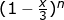Question

If the first 3 terms of the expansion of are , find the value of x and n

Solution

Recall the binomial expansion formula

Next we need to simplify

Going back to the question,

We can then form 2 equations involving n and x

Equation 1

Equation 2

### Integration of Trig Functions Part II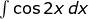Be careful of the negative signs when integrating sin and cos functions

Question 1
a)

Note the absence of the negative sign
We divide by 2 as the differentiation of 2x is 2

b)

Note the presence of the negative sign
We divide by 2 as the differentiation of 2x is 2

Question 2
a)

Note the presence of the negative sign

b)

Note the absence of the negative sign